Next: Conduction electrons in a Up: Quantum statistics Previous: Black-body radiation

## The Stefan-Boltzmann law

The total power radiated per unit area by a black-body at all frequencies is given by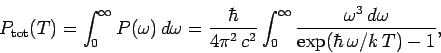(653)

or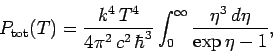(654)

where. The above integral can easily be looked up in standard mathematical tables. In fact,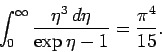(655)

Thus, the total power radiated per unit area by a black-body is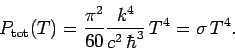(656)

Thisdependence of the radiated power is called the Stefan-Boltzmann law, after Josef Stefan, who first obtained it experimentally, and Ludwig Boltzmann, who first derived it theoretically. The parameter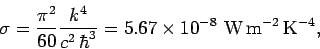(657)

is called the Stefan-Boltzmann constant.

We can use the Stefan-Boltzmann law to estimate the temperature of the Earth from first principles. The Sun is a ball of glowing gas of radius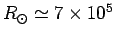km and surface temperatureK. Its luminosity is(658)

according to the Stefan-Boltzmann law. The Earth is a globe of radiuskm located an average distance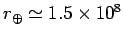km from the Sun. The Earth intercepts an amount of energy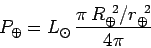(659)

per second from the Sun's radiative output: i.e., the power output of the Sun reduced by the ratio of the solid angle subtended by the Earth at the Sun to the total solid angle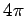. The Earth absorbs this energy, and then re-radiates it at longer wavelengths. The luminosity of the Earth is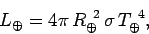(660)

according to the Stefan-Boltzmann law, where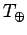is the average temperature of the Earth's surface. Here, we are ignoring any surface temperature variations between polar and equatorial regions, or between day and night. In steady-state, the luminosity of the Earth must balance the radiative power input from the Sun, so equatingandwe arrive at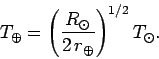(661)

Remarkably, the ratio of the Earth's surface temperature to that of the Sun depends only on the Earth-Sun distance and the solar radius. The above expression yields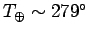K or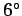C (or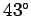F). This is slightly on the cold side, by a few degrees, because of the greenhouse action of the Earth's atmosphere, which was neglected in our calculation. Nevertheless, it is quite encouraging that such a crude calculation comes so close to the correct answer.Next: Conduction electrons in a Up: Quantum statistics Previous: Black-body radiation
Richard Fitzpatrick 2006-02-02